#DAY 28
1

## Day28 - SVG filter - feImage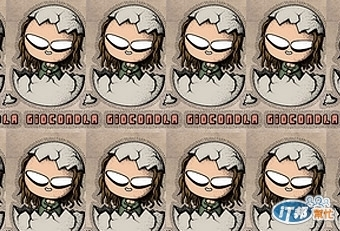feImage 是 SVG filter 裏頭最簡單的濾鏡，不過為什麼要用一篇文章專門介紹它呢？就是因為 feImage 可以幫助我們更實際的了解 filter 的原理，除此之外，這篇文章也會搭配另外一個最簡單的濾鏡 feTile ( 到底有幾個最簡單呀 ) 藉由實際的範例來深入了解 SVG filter 的博大精深吧！( oxxostudio.tw 同步發表：SVG 研究之路 (27) - filter - feImage )

feImage 人如其名的就是把圖片當濾鏡，設定圖片的長寬，搭配 feTitle 就可以把圖片重複運用在我們的圖形當中，有點類似 SVG Patterns，一如往常，先來瞧瞧 feImage 有哪些屬性可以設定：
2. x
3. y
4. width
5. height
6. preserveAspectRatio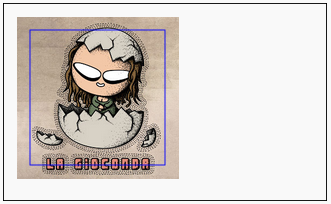``````<svg width="360" height="220">
<defs>
<filter id="f">
</filter>
</defs>
<rect x="30" y="30" width="150" height="150" filter="url(#f)" />
<rect x="30" y="30" width="150" height="150" stroke="#00f" fill="none" />
<rect x="0" y="0" width="360" height="220" stroke="#000" fill="none" stroke-width="2" />
</svg>
``````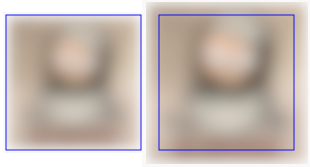``````  <defs>
<filter id="f">
<feImage xlink:href="http://www.oxxostudio.tw/img/articles/201410/20141003_1_demo.jpg" x="0" y="0" width="100" height="100" />
</filter>
</defs>
<rect x="30" y="30" width="150" height="150" filter="url(#f)" />
<rect x="30" y="30" width="150" height="150" stroke="#00f" fill="none" />
``````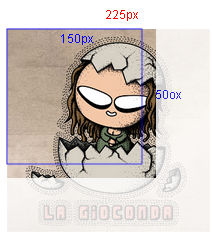``````  <defs>
<filter id="f" primitiveUnits="objectBoundingBox">
<feImage xlink:href="http://www.oxxostudio.tw/img/articles/201410/20141003_1_demo.jpg" x="0" y="0" width="1.5" height="1.5" />
</filter>
</defs>
<rect x="30" y="30" width="150" height="150" filter="url(#f)" />
<rect x="30" y="30" width="150" height="150" stroke="#00f" fill="none" />
````````````  <defs>
<filter id="f" primitiveUnits="objectBoundingBox" x="0" y="0" width="1" height="1">
<feImage xlink:href="http://www.oxxostudio.tw/img/articles/201410/20141003_1_demo.jpg" x="0" y="0" width="1.5" height="1.5" />
</filter>
</defs>
<rect x="30" y="30" width="150" height="150" filter="url(#f)" />
<rect x="30" y="30" width="150" height="150" stroke="#00f" fill="none" />
````````````  <defs>
<filter id="f" primitiveUnits="objectBoundingBox">
<feImage xlink:href="http://www.oxxostudio.tw/img/articles/201410/20141003_1_demo.jpg" x="0" y="0" width="1" height="1" />
</filter>
</defs>
<rect x="30" y="30" width="150" height="150" filter="url(#f)" />
<rect x="30" y="30" width="150" height="150" stroke="#00f" fill="none" />
``````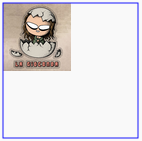``````<feImage xlink:href="http://www.oxxostudio.tw/img/articles/201410/20141003_1_demo.jpg" x="0" y="0" width=".5" height=".5" />
````````````  <defs>
<filter id="f" primitiveUnits="objectBoundingBox" x="0" y="0" width="1" height="1">
<feImage xlink:href="http://www.oxxostudio.tw/img/articles/201410/20141003_1_demo.jpg" width="1" height="1" preserveAspectRatio="xMidYMax slice" />
</filter>
</defs>
<rect x="30" y="30" width="250" height="150" filter="url(#f)" />
<rect x="30" y="30" width="250" height="150" stroke="#00f" fill="none" />
````````````<feImage xlink:href="http://www.oxxostudio.tw/img/articles/201410/20141003_1_demo.jpg" width=".2" height="1" preserveAspectRatio="xMaxYMax slice" />
````````````  <defs>
<filter id="f" primitiveUnits="objectBoundingBox" x="0" y="0" width="1" height="1">
<feTile/>
</filter>
</defs>
<rect x="30" y="30" width="250" height="150" filter="url(#f)" />
<rect x="30" y="30" width="250" height="150" stroke="#00f" fill="none" />
``````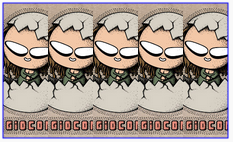``````<feImage xlink:href="http://www.oxxostudio.tw/img/articles/201410/20141003_1_demo.jpg" width=".2" height="1" preserveAspectRatio="xMidYMax slice"/>
<feTile/>
``````

SVG 30 天 ~ 就要醬玩31